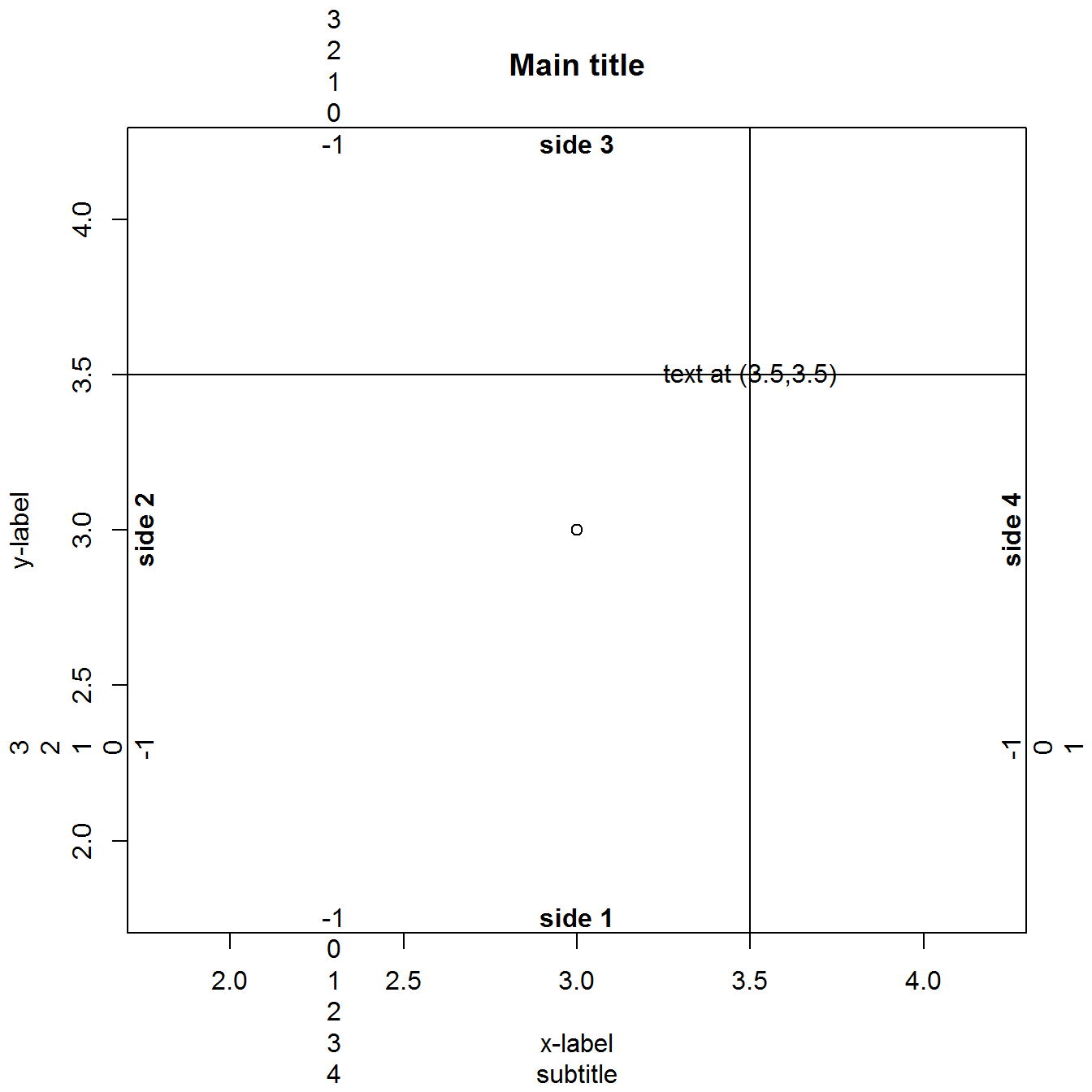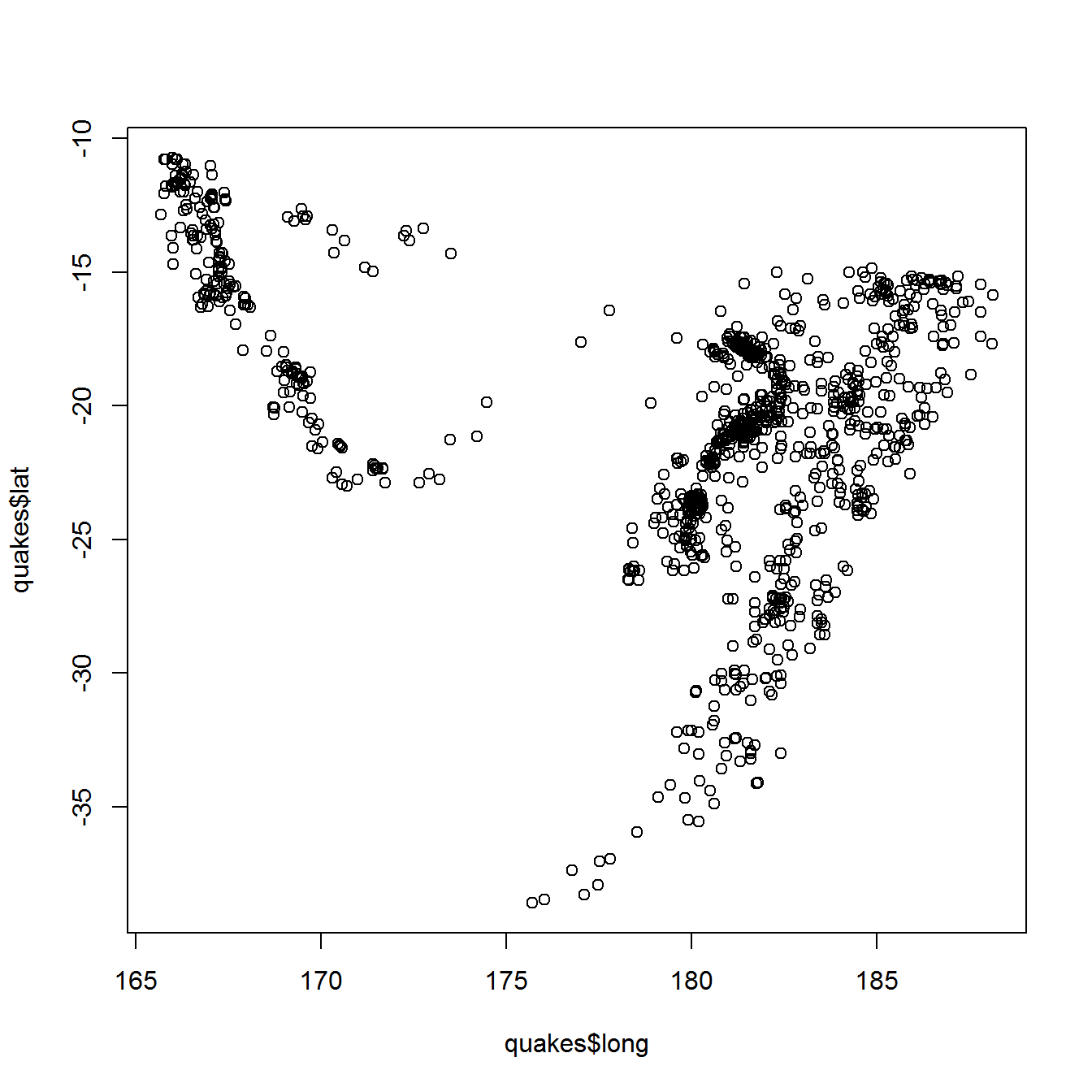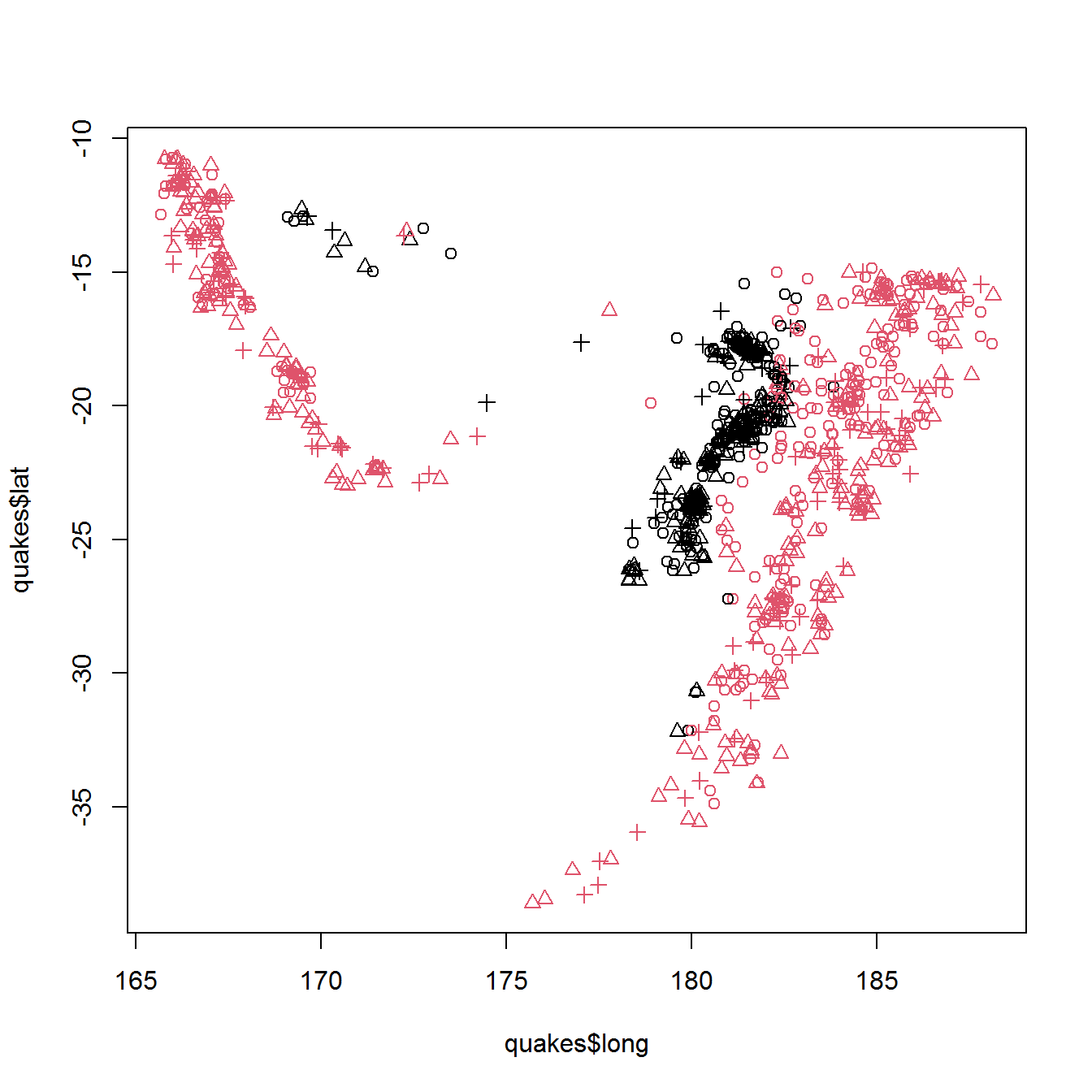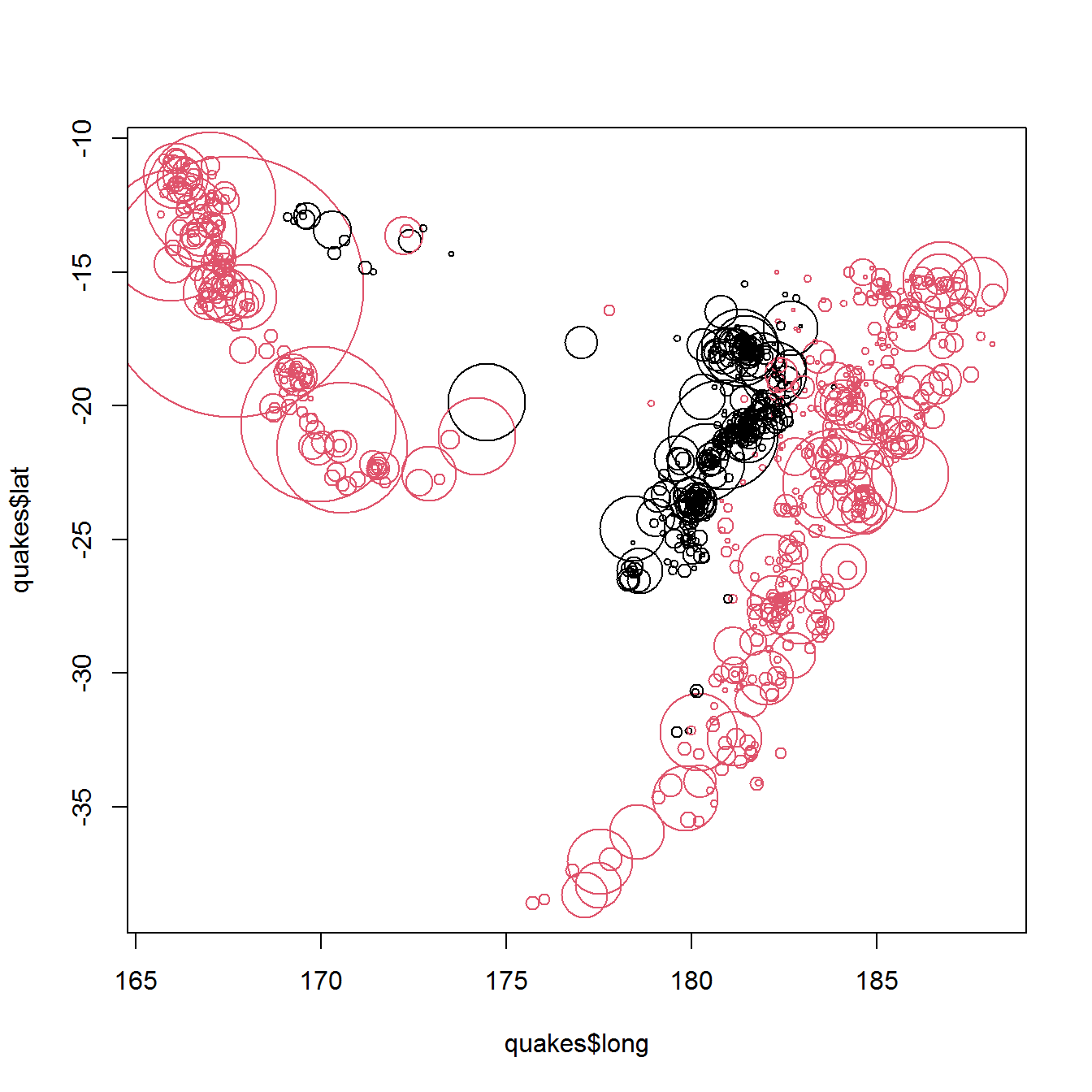The objective of these computer exercises is to become familiar with the basic structure of R and to learn some more sophisticated tips and tricks of R. The R code to perform the analyses, together with the output, is given in a separate file. However, you are strongly advised to write the code yourself. Questions are posed to reflect on what you are doing and to study output and results in more detail.

# 1 Basics

If you have not done so already, start R via Starten - Alle programma’s - R - R 4.3.0 (older versions of R should also be fine). This opens the R console. R is a command line driven environment. This means that you have to type in commands (line-by-line) for it to compute or calculate something. In its simplest form R can therefore be used as a pocket calculator:

2+2
#  4

Question 1. (a) Look up today’s exchange rate of the euro versus the US$on the Internet. Use R to calculate how many US$ is 15 euro.

(b) Round the number you found to the second decimal using function round (use help or ? to inspect the arguments of the function) and assign the result to an object curr.

(c) Use mode, str, and summary to inspect the object you created. These functions give a compact display of the type of object and its contents.

Luckily R is more than just a calculator, it is a programming language with most of the elements that every programming language has: statements, objects, types, classes etc.

# 2 R syntax: vectors

Question 2. (a) One of the simplest data structures in R is a vector. Use the function seq to generate a vector vec that consists of all numbers from 11 to 30 (see ?seq for documentation).

(b) Select the 7th element of the vector.

(c) Select all elements except the 15th.

(d) Select the 2nd and 5th element of the vector.

(e) Select only the odd valued elements of the vector vec. Do this is in two steps: first create the appropriate vector of indices index using the function seq, then use index to make the selection.

Until now you have only used the R console. However, RStudio (Starten - Alle programma’s - R - RStudio) provides a much nicer and richer interface when working with R. Although all exercises can be made within the basic R environment, we highly recommend to use RStudio.

Question 3. (a) What are the windows that RStudio consists of?

From now on we advise you to use RStudio. You can use either the Console or the Script window (the upper left window). We recommend you to use the Script window, since this allows you to easily save your code to a file for later usage.

(b) Use the elementary functions /, -, ^ and the functions sum and length to calculate the mean $$\bar{x}=\sum_i x_i/n$$ and the standard deviation $$\sqrt{\sum_i(x_i-\bar{x})^2/(n-1)}$$ of the vector vec of all numbers from 11 to 30. You can verify your answer by using the built-in R functions mean and sd.

(c) Once you completed the analysis, have a look at the Environment and History windows. Do you understand their contents?

Question 4. R comes with many predefined datasets. You can type data() to get the whole list. The islands dataset gives the areas of the world’s major landmasses exceeding 10,000 square miles. It can be loaded by typing:

# This could in this case actually be skipped since the package datasets is
data(islands)

(a) Inspect the object using the functions head, str etc. Also have a look at the help file using help(islands).

(b) How many landmasses in the world exceeding 10,000 square miles are there?

(c) Make a Boolean vector that has the value TRUE for all landmasses exceeding 20,000 square miles.

(d) Select the islands with landmasses exceeding 20,000 square miles.

(e) Make a character vector that only contains the names of the islands.

(f) The Moluccas have mistakenly been counted as a single island. The largest island of the Moluccas, Halmahera, only has about 7,000 square miles. Remove Moluccas from the data. Hint: an elegant solution uses the Boolean operator !=.

# 3 R syntax: matrices

Question 5. (a) Create a character matrix M with 6 rows and three columns containing the first eighteen letters of the Roman alphabet (hint: see ?letters). Fill the matrix column-wise.

(b) Which is the letter on the fifth row and second column?

(c) Replace the letters in the last column of M with the last six letters of the Roman alphabet.

(d) Make a character vector containing all the vowels and use this vector to replace the vowels in M with the character string “vowel”.

(e) Now replace all consonants in M with the character string “consonant”.

(f) Count the number of elements in M that are equal to “consonant” and “vowel” respectively.

# 4 Data frames, data import, missing values and factors

Question 6. We are going to use yet another predefined dataset: mtcars. These data were extracted from the 1974 Motor Trend US magazine, and comprise fuel consumption and 10 aspects of automobile design and performance for 32 automobiles (1973–74 models). See ?mtcars for the definition of the variables contained in it.

(a) What kind of data structure is the mtcars data?

(b) How many car models can ride more than 25 miles per gallon?

(c) What are the names of the car models that can ride more than 25 miles per gallon?

(d) Select the car models that can ride less than 22 miles per gallon and have more than 6 cylinders.

(e) In addition to the previous selection, also select the car models that either have more than 3 carburetors or weigh less than 3500 lbs. How many car models satisfy these conditions?

(f) The variable am is a numeric variable that indicates the type of transmission:

• 0: automatic
• 1: manual

Add a variable called am_char to the mtcars data set where you recode the variable am and replace 0 by “automatic” and 1 by “manual”.

We will work with a data set that gives the survival status of passengers on the Titanic. See titanic3info.txt for a description of the variables. Some background information on the data can be found on titanic.html.

Question 7. (a) Download the titanic data set titanic3.dta in STATA format from the course website and import it into R using the function read.dta. Do not use the function read_dta from the haven package nor the ‘Import Dataset’ option in RStudio. This leads to slight differences in the imported data which may lead to problems in some of the exercises. Give the data set an appropriate name as an R object. E.g., we can call it titanic3 (but feel free to give it another name). Before importing the data, you first have to load the foreign package in which the function read.dta is defined. Moreover, you probably will need to point R to the right folder where you saved the file titanic3.dta. You can do this by changing the so-called working directory, which will be explained later. Using the basic R environment you can do this via the menu (File - Change dir), and similarly for RStudio (Session - Set Working Directory - Choose Directory).

We take a look at the values in the titanic data set.

(b) First, make the whole data set visible in a spreadsheet like format (how this is done depends on whether base R or RStudio is used).

(c) Often, it is sufficient to show a few records of the total data set. This can be done via selections on the rows, but another option is to use the functions head and tail. Use these functions to inspect the first 4 and last 4 records (instead of 6 which is the default).

The function dim can be used to find the number of rows (passengers in this case) and columns (variables) in the data.

dim(titanic3)

An alternative is the function str. This function shows information per column (type of variable, first records, levels in case of factor variables) as well as the total number of rows and columns.

str(titanic3)

(d) Issue both commands and study the output.

(e) Summarize the data set by using the summary function.

This gives a summary of all the variables in the data set. We can see that for categorical variables like pclass a frequency distribution is given, whereas for continuous variables like age numerical summaries are given. Still, for some variables we do not automatically get what we would like or expect:

• The variable survived is dichotomous with values zero and one, which are interpreted as numbers.
• The categorical variable pclass is represented differently from the categorical variable home.dest.
• The variable dob, which gives the date of birth, is represented by large negative numbers.

In subsequent exercises, we will shed further light on these anomalies and we will try to repair some of these.

Question 8. We can also summarize specific columns (variables) of a data.frame. There are many ways to summarize a variable, depending on its structure and variability. For continuous variables, the same summary function can be used, but other options are the functions mean, quantile, IQR, sd and var. For categorical summaries, one may use summary and table. Note that missing values are treated differently depending on the function used.

(a) Summarize the age variable in the titanic data set (the age column is selected via titanic3$age). Give the 5%, 25%, 50%, 75% and 95% quantiles and the inter-quartile range. You may have to take a look at the help files for the functions. (b) Summarize the survived variable using the table function. Also give a two-by-two table for sex and survival status using the same table function. Question 9 (OPTIONAL). Instead of the file in STATA format, we also made available part of the titanic data set in tab-delimited format (titanic3select.txt). Download this file from the course website and then import it using the command read.table. Note that this file has been manipulated in Excel and that importing the data is not as straightforward as you would have hoped. You will need to tweak the arguments of read.table and/or make some changes to the file manually. Question 10. We have a further look at the output from the summary function, which was not always what we would like to see. First, give the commands (sapply will be explained later): sapply(titanic3, mode) # pclass survived name sex age sibsp # "numeric" "numeric" "character" "numeric" "numeric" "numeric" # parch ticket fare cabin embarked boat # "numeric" "character" "numeric" "numeric" "numeric" "numeric" # body home.dest dob family agecat # "numeric" "character" "numeric" "numeric" "numeric" sapply(titanic3, is.factor) # pclass survived name sex age sibsp parch ticket # TRUE FALSE FALSE TRUE FALSE FALSE FALSE FALSE # fare cabin embarked boat body home.dest dob family # FALSE TRUE TRUE TRUE FALSE FALSE FALSE TRUE # agecat # TRUE We see that survived has mode “numeric” and is not a factor (is.factor(titanic3$survived) gives FALSE). It is interpreted in the same way as the truly continuous variable age.

(a) Add a variable status to the titanic data set, which gives the survival status of the passengers as a factor variable with labels “no” and “yes” describing whether individuals survived. Make the value “no” the first level (see titanic3info.txt for the reason).

(b) Variable pclass has mode “numeric” and is a factor, whereas home.dest has mode “character” and is not a factor. Give the commands

as.numeric(head(titanic3$pclass)) as.numeric(head(titanic3$home.dest))

and explain the difference in output.

We do not change the variables in this dataset that have mode “character”. They are variables that have many different values, and there is no reason to convert them into factors.

Question 11. (a) Take a look at the name (name), and the home town/destination (home.dest) of all passengers who were older than 70 years. Use the appropriate selection functions.

(b) There is one person from Uruguay in this group. Select the single record from that person. Did this person travel with relatives?

(c) Make a table of survivor status by sex, but only for the first class passengers. Use the xtabs function.

# 5 Graphics

## 5.1 Basic graphics

The graphics subsystem of R offers a very flexible toolbox for high-quality graphing. There are typically three steps to producing useful graphics:

• Creating the basic plot
• Enhancing the plot with labels, legends, colors etc.
• Exporting the plot from R for use elsewhere

In the graphics model that R uses, a figure region consists of a central plotting region surrounded by margins. The basic graphics command is plot. The following piece of code illustrates the most common options:

plot(3,3,main="Main title",sub="subtitle",xlab="x-label",ylab="y-label")
text(3.5,3.5,"text at (3.5,3.5)")
abline(h=3.5,v=3.5)
for (side in 1:4) mtext(-1:4,side=side,at=2.3,line=-1:4)
mtext(paste("side",1:4),side=1:4,line=-1,font=2)In this figure one can see that

• Sides are labelled clockwise starting with x-axis
• Coordinates in the margins are specified in lines of text
• Default margins are not all wide enough to hold all numbers -1,…,4

You might want to use the help function to investigate some of the other functions and options.

Plots can be further finetuned with the par function. For instance, the default margin sizes can be changed using par. The default settings are

par("mar")
#  5.1 4.1 4.1 2.1

This explains why only side 1 in the figure had a wide enough margin. This can be remedied by setting

par(mar=c(5,5,5,5))

before plotting the figure.

Question 12. (a) Try making this figure yourself by executing the code shown above.

(b) Save the figure you just made to a file. For this you have to know that R sends graphics to a device. The default device on most operating systems is the screen, for example the “Plots” window in RStudio. Saving a figure, therefore, requires changing R’s current device. See help(device) for the options. Save the figure you just made to a png and a pdf file. Don’t forget to close the device afterwards.

(c) When saving a figure to file, default values for the figure size are used. Save the figure to a pdf file with width and height of 21 inches.

Question 13. The quakes data set gives location and severity of earthquakes off Fuji. First load the data:

data(quakes)

(a) Make a scatterplot of latitude versus longitude. You should obtain a graph similar to:(b) Use cut to divide the magnitude of quakes into three categories (cutoffs at 4.5 and 5) and use ifelse to divide depth into two categories (cutoff at 400). Hint: have a careful look at the (admittedly complicated) help page of cut, in particular the arguments breaks and include.lowest.

(c) Redraw the plot, with symbols by magnitude and colors by depth. You should obtain a graph similar to:(d) The magnitude of the earthquakes is given in Richter scale. Calculate the energy released in each earthquake as $$10^{(3/2)}$$ to the power of the Richter measurement.

(e) The cex argument of plot can be used to scale the plot symbols. We will scale the plot symbols so that the surface of the plot symbol is proportional to the released energy. Calculate plot symbol size as the square root of the energy divided by the median square root of the energy (to get median symbol size 1).

(f) Plot the magnitude of earthquakes again, but with plot symbols sized by energy. Keep the coloring by depth. You should obtain a graph similar to:Question 14. Different plots can be combined in a single window (device) via , e.g., par(mfrow=c(..)) or layout(..). Combine the histogram and the two boxplots for the titanic data from the lecture into a single plot. Find a nice layout of your final plot, see help files for setting proper margins, etc. You should obtain a graph similar to: